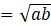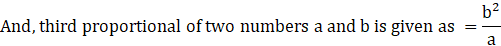Question of The Day06-07-2020

What will be the ratio of the mean proportional of 5.4 and 0.6 to the third proportional of 12 and 4.8?

Correct Answer : b ) 15 : 16

Explanation :

We know that

Mean proportional of two numbers a and b is given asThus, mean proportional of 5.4 and 0.6 is

$$\sqrt{5.4*0.6}=\sqrt{3.24}=1.8$$

And, the third proportional of 12 and 4.8 is

$${4.8*4.8 \over 12}=1.92$$

Therefore,

Required ratio = 1.8 : 1.92 = 15 : 16

Hence, (b) is the correct answer.

Such type of question is asked in various government exams like SSC CGL, SSC MTS, SSC CPO, SSC CHSL, RRB JE, RRB NTPC, RRB GROUP D, RRB OFFICER SCALE-I, IBPS PO, IBPS SO, RRB Office Assistant, IBPS Clerk, RBI Assistant, IBPS RRB OFFICER SCALE 2&3, UPSC CDS, UPSC NDA etc.

Read Daily Current Affairs, Banking Awareness, Hindi Current Affairs, Word of the Day, and attempt free mock tests at PendulumEdu and boost your preparation for the actual exam.0